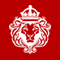##### Profit with a clickFinancika

Free Mobile App

## The Moving Averages

Most chart patterns show many fluctuations in price movement. In order to smooth out the price movements, traders apply moving averages. A moving average is the average price of a security over a specified period.

The method can be used to identify whether a security or the market is moving uptrend or downtrend.

Once the price fluctuations are smoothed, traders can analyze trends easier and make better decisions.

● Simple Moving Average

This is the easiest and most commonly used method. When using simple moving average, traders take the sum of all of the past closing prices over the period and divide the result by the number of prices used in the calculation. For instance, in a 10-day moving average, the last 10 closing prices are added together and then divided by 10.

● Linear Weighted Average

Linear weighted moving average is calculated by taking the sum of all closing prices over a period, multiplying them by the position of the data point and then dividing by the sum of the number of periods.

● Exponential Moving Average

Exponential moving average calculation places a higher weight on recent data points. It is not vital for traders to understand the calculation method, because most charting packages perform the calculation. This method is more responsive to new types of information compared to the Simple Moving Average method.

Unfortunately, we do not offer our services to residents.

Our apologies, but we cannot serve clients from this country at this time.

Our apologies, but we cannot serve clients from this country at this time.

OK